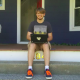author David ThompsonMon, 7 Jan 2019 04:19:46 +0000 (23:19 -0500) committer David ThompsonMon, 7 Jan 2019 04:19:46 +0000 (23:19 -0500)

index fe1a681..252f368 100644 (file)
@@ -1,5 +1,6 @@
+             (chickadee render color)
@@ -23,6 +24,9 @@
(right-stick ,(vec2 450.0 20.0) 13 31)
(left-shoulder ,(vec2 110.0 390.0) 4 22)
(right-shoulder ,(vec2 450.0 390.0) 5 23)))
(right-stick ,(vec2 450.0 20.0) 13 31)
(left-shoulder ,(vec2 110.0 390.0) 4 22)
(right-shoulder ,(vec2 450.0 390.0) 5 23)))
+(define trigger-icons
+  `((trigger-left ,(vec2 10.0 390.0) 22)
+    (trigger-right ,(vec2 550.0 390.0) 23)))
(define no-controller-msg "no controller detected   :'(")
(define no-controller-msg-pos #f)
(define controller-name-pos #f)
(define no-controller-msg "no controller detected   :'(")
(define no-controller-msg-pos #f)
(define controller-name-pos #f)
(set! controller-name-pos
(vec2 (center-text (controller-name controller)) 440.0)))

(set! controller-name-pos
(vec2 (center-text (controller-name controller)) 440.0)))

-;; FIXME: I couldn't think of a decent way to display the analog
-;; left/right trigger buttons, so they are omitted.
(define (draw alpha)
(if controller
(define (draw alpha)
(if controller
-      (begin
-        (draw-text (controller-name controller) controller-name-pos)
-        (for-each (match-lambda
-                    (((and (or 'left-stick 'right-stick) button)
-                      p released pressed)
-                     (let ((pressed? (controller-button-pressed? controller
-                                                                 button))
-                           (x (controller-axis controller
-                                               (if (eq? button 'left-stick)
-                                                   'left-x
-                                                   'right-x)))
-                           (y (controller-axis controller
-                                               (if (eq? button 'left-stick)
-                                                   'left-y
-                                                   'right-y))))
-                       (draw-sprite (texture-atlas-ref atlas released)
-                                    p)
-                       (when (or pressed? (not (zero? x)) (not (zero? y)))
-                         (draw-sprite (texture-atlas-ref atlas pressed)
-                                      (vec2+ p (vec2 (* x 16.0) (* y -16.0)))))))
-                    ((button p released pressed)
-                     (let ((pressed? (controller-button-pressed? controller
-                                                                 button)))
-                       (draw-sprite (texture-atlas-ref
-                                     atlas
-                                     (if pressed? pressed released))
-                                    p))))
-                  button-icons))
+      (with-batched-sprites
+       (draw-text (controller-name controller) controller-name-pos)
+       (for-each (match-lambda
+                   ((axis p tile)
+                    (let ((alpha (controller-axis controller axis)))
+                      (draw-sprite (texture-atlas-ref atlas tile) p
+                                   #:tint (color 1.0 1.0 1.0 alpha)))))
+                 trigger-icons)
+       (for-each (match-lambda
+                   (((and (or 'left-stick 'right-stick) button)
+                     p released pressed)
+                    (let ((pressed? (controller-button-pressed? controller
+                                                                button))
+                          (x (controller-axis controller
+                                              (if (eq? button 'left-stick)
+                                                  'left-x
+                                                  'right-x)))
+                          (y (controller-axis controller
+                                              (if (eq? button 'left-stick)
+                                                  'left-y
+                                                  'right-y))))
+                      (draw-sprite (texture-atlas-ref atlas released)
+                                   p)
+                      (when (or pressed? (not (zero? x)) (not (zero? y)))
+                        (draw-sprite (texture-atlas-ref atlas pressed)
+                                     (vec2+ p (vec2 (* x 16.0) (* y -16.0)))))))
+                   ((button p released pressed)
+                    (let ((pressed? (controller-button-pressed? controller
+                                                                button)))
+                      (draw-sprite (texture-atlas-ref
+                                    atlas
+                                    (if pressed? pressed released))
+                                   p))))
+                 button-icons))
(draw-text no-controller-msg no-controller-msg-pos)))